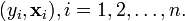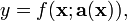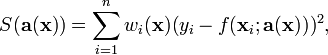# Moving least squares

Moving least squares is a method of approximating a continuous function from a set of eventually unorganized point samples via the calculation of a weighted least squares measure biased towards the region around the point at which the approximation value is requested.

In computer graphics, the moving least squares method is useful for reconstructing a surface from a set of points. Often it is used to create a 3D surface from a cloud of points through either downsampling or upsampling.

## Problem statement

Consider the problem of adjusting an approximation of some function to best fit a given data set. The data set consist of n points$(y_i,\bold{x}_i), i = 1, 2,\dots, n .$

We define an approximation in a similar way as in the weighted least squares, but in such a way that its adjustable coefficients depend on the independent variables:$y=f(\bold{x};\bold{a}(\bold{x})) ,$

where y is the dependent variable, x are the independent variables, and a(x) are the non-constant adjustable parameters of the model. In each point x where the approximation should be evaluated, we calculate the local values of these parameters such that the model best fits the data according to a defined error criterion. The parameters are obtained by minimization of the weighted sum of squares of errors,$S(\bold{a}(\bold{x})) = \sum_{i=1}^n w_i(\bold{x}) (y_i - f(\bold{x}_i;\bold{a}(\bold{x})))^2 ,$

with respect to the adjustable parameters of the model a(x) in the point of evaluation of the approximation. Note that weights are replaced by weighting functions, which are usually bell-like functions centered around xi.Some content on this page may previously have appeared on Citizendium.